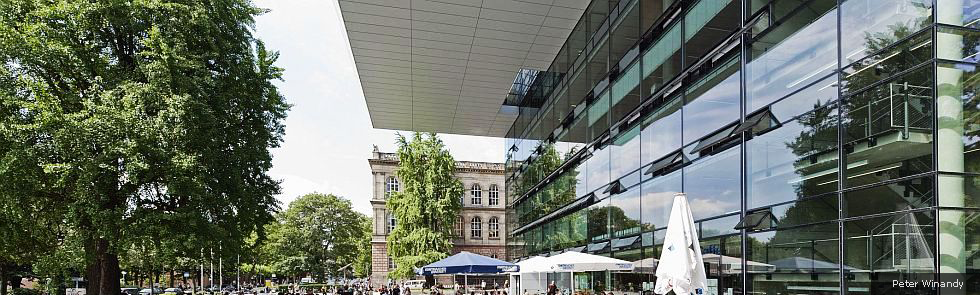Kategorien
Seiten
-Numerical Reconstruction Techniques for the Boltzmann Equation

09. Oktober 2018 | vonProf. Dr. Manuel Torillhon

Rarefied gas flows, or equivalently flows in microscopic settings, are one of the most prominent examples of flow processes where classical models of thermodynamics fail and enhanced non-equilibrium models become mandatory for simulations. This requires to consider the probability density of the particle velocities in the context of kinetic gas theory an Boltzmann equation. This project has several aims.
There is need to develop a hybrid reconstruction technique for the probability density combining the context of moment models, discrete velocity schemes and direct simulation Monte-Carlo in order to switch between the methods whatever is optimal. Another related challenge is the efficient evaluation of the Boltzmann collision operator. These aspects must be combined in numerical experiments based on the new technique for near-vacuum and supersonic flows.

Kommentare sind geschlossen.